Practice the questions of McGraw Hill Math Grade 8 Answer Key PDF Lesson 21.3 Circles to secure good marks & knowledge in the exams.

Exercises

SOLVE

Question 1.
What is the diameter of the circle?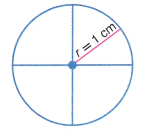The diameter of the circle is equal to 2 cms,

Explanation:
As we know the diameter of the circle is equal to 2 X radius, so as the given circle has
r = 1 cm so diameter = 2 x 1 cm = 2 cms.

Question 2.
What is the diameter of the circle?The diameter of the circle is equal to 14 in.,

Explanation:
As we know the diameter of the circle is equal to 2 X radius, so as the given circle has
r = 1 cm so diameter = 2 x 7 in. = 14 inches.

Question 3.
What is the radius of the circle below?The radius of the circle is equal to 4 ft,

Explanation:
As we know the diameter of the circle is equal to 2 X radius, so as the given circle has
d = 8 ft so radius = diameter/ 2 = 8 ft/2 = 4 ft.

Question 4.
What is the center of the circle?The center of the circle is d/2,

Explanation:
As we know the diameter of the circle is equal to 2 X radius so as the given circle has
diameter = 2 x radius or center, therefore center = d/2.

Question 5.
What is the radius of the circle?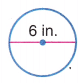The radius of the circle is equal to 3 in.,

Explanation:
As we know the diameter of the circle is equal to 2 X radius, so as the given circle has
d = 6 in. so radius = diameter/ 2 = 6 in/2 = 3 in.

Question 6.
What are the two radii of the circle?OA,OB,

Explanation:
As a Radius or Radii of a circle is the distance from the center of the circle to any point on it’s circumference. So the given circle has two radii they are OA and OB.

SOLVE

Question 1.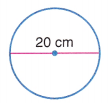What is the area of the circle? ___________
What is the circumference? ___________
The area of the circle is 314.285 cm2,
The circumference of the circle is 62.857 cm,

Explanation:
Given the diameter of the circle as 20 cm as we know the diameter of the circle is equal to 2 X radius, so as the given circle has d = 20 cm so radius = diameter/ 2 = 20 cm/2 = 10 cm. We know area of the circle as A = π r², A = 3.14285 X 10 cm X 10 cm = 314.285 cm2 and circumference of the circle is C = 2πr = 2 X 3.14285 X 10 cm = 62.857 cm.

Question 2.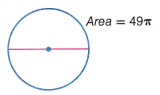What is the circumference of the circle? ___________
The circumference of the circle is 14 π,

Explanation:
Given the area of the circle as 49π as we know area of the circle as A = π r²,
49 π = π X r X r, So r= square root of 49 so r = 7 and as circumference of the circle is C = 2πr = 2 X π X 7 = 14π.

Question 3.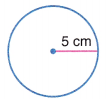What is the diameter of the circle? ___________
What is the area? ___________
What is the circumference? ___________
The diameter of the circle is equal to 10 cm,
The area of the circle is 78.57125 cm2,
The circumference of the circle is 31.4285 cm,

Explanation:
As we know the diameter of the circle is equal to 2 X radius given r = 5 cm so as the given circle has d = 2 X 5 cm = 10 cm. As we know area of the circle as A = π r², A = 3.14285 X 5 cm X 5 cm = 78.57125 cm2 and circumference of the circle is C = 2πr = 2 X 3.14285 X 5 cm = 31.4285 cm.

Question 4.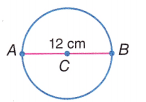What is the circumference of the circle? ___________
What is the area of the circle? ___________
The circumference of the circle is 37.7142 cm,
The area of the circle is  113.1426 cm2,

Explanation:
As we know the diameter of the circle is equal to 2 X radius given d = 12 cm so as the given circle has r = 12/2 cm = 6 cm. As we know circumference of the circle is C = 2πr = 2 X 3.14285 X 6 cm = 37.7142 cm and area of the circle as A = π r², A = 3.14285 X 6 cm X 6 cm = 113.1426 cm2.

Question 5.
A bicycle wheel measures 25 inches in diameter. How far would a bike travel if the wheel went completely around 4 times?
____________ inches
Far would a bike travel if the wheel went completely around 4 times is 78.57125 inches,

Explanation:
Given A bicycle wheel measures 25 inches in diameter. Far would a bike travel if the wheel went completely around 4 times as we know the diameter of the circle is equal to 2 X radius given d = 25 inches so as the given circle has r = 25/2 inches = 12.5 inches. As we know circumference of the circle is C = 2πr = 2 X 3.14285 X 12.5 inches = 78.57125 inches.

Question 6.
Brenda walks her dog along two circular routes laid out in the park. How much longer is Route B then route A? (Leave the answer in terms of pi.)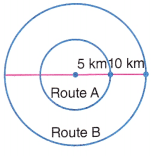_____________ π km